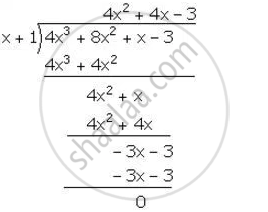Share

Books Shortlist

# The expression 4x3 – bx2 + x – c leaves remainders 0 and 30 when divided by x + 1 and 2x – 3 respectively. Calculate the values of b and c. Hence, factorise the expression completely. - ICSE Class 10 - Mathematics

ConceptFactorising a Polynomial Completely After Obtaining One Factor by Factor Theorem

#### Question

The expression 4x3 – bx2 + x – c leaves remainders 0 and 30 when divided by x + 1 and 2x – 3 respectively. Calculate the values of b and c. Hence, factorise the expression completely.

#### Solution

Let f (x)=4x^3-bx^2+x-c

it is given that when f(x) is dividend by (x+1), the remainder is 0

∴ f(-1)=0

4(-1)^3-b(1)^2+(-1)-c=0

-4-b-1-c=0

b+c+5=0 ........(1)

It is given that when f(x) is divided by (2x-3) the remainder is 30.

∴ f(3/2)=30

4(3/2)^3 -b(3/2)^2+(3/2)-c =30

27/2-(9b)/4+3/2-c=30

54-9b+6-4c-120=0

9b+4c+60=0   .............(2)

Multiplying (1) by 4 and subtracting it from (2), we get,

5b+40=0

b=-8

Substituting the value of b in (1), we get,

c=-5+8=3

Therefore, f(x) =4x^3+8x^x-3

Now, for x-1, wr get,

f(x)=f(-1)=4(-1)^3+8(-1)^2+(-1)-3=-4+8-1-3=0

Hence, (x+1) is a factor of f (x)∴ 4x^3+8x^2+x-3=(x+1)(4x^2+4x-3)

=(x+1)(4x^2+6x-2x-3)

=(x+1)[2x(2x+3)-(2x+3)]

= (x+1)(2x+3)(2x-1)

Is there an error in this question or solution?

#### APPEARS IN

Solution The expression 4x3 – bx2 + x – c leaves remainders 0 and 30 when divided by x + 1 and 2x – 3 respectively. Calculate the values of b and c. Hence, factorise the expression completely. Concept: Factorising a Polynomial Completely After Obtaining One Factor by Factor Theorem.
S